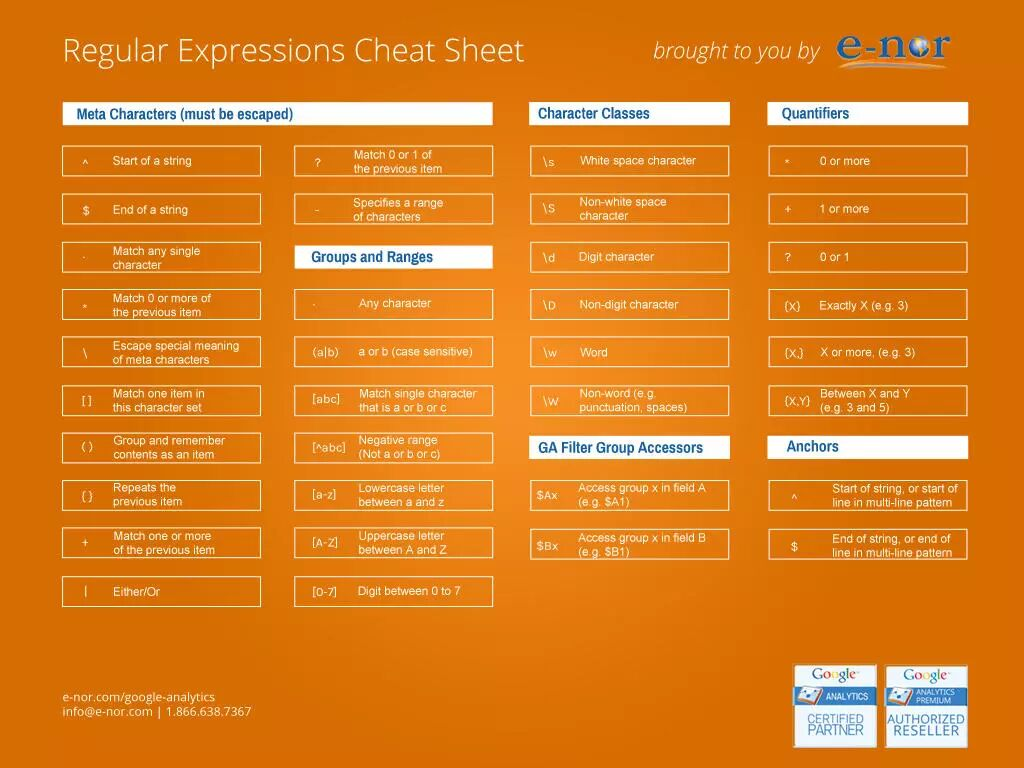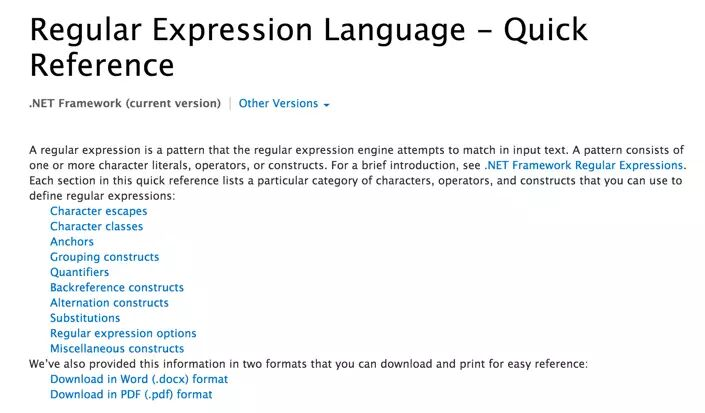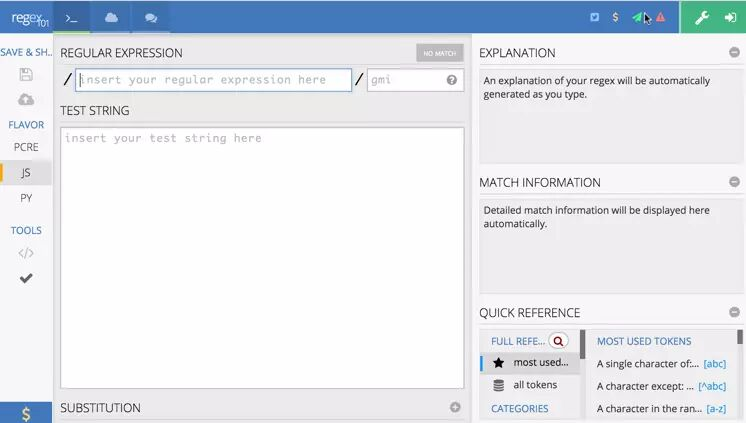# 20个前端开发中常用的正则表达式

``var reg = /^(\\d{1,4})(-|\\/)(\\d{1,2})\\2(\\d{1,2})\$/; ``var r = fieldValue.match(reg);             ``if(r==null)alert('Date format error!');``

#### 1 、 校验密码强度

``^(?=.*\\d)(?=.*[a-z])(?=.*[A-Z]).{8,10}\$``

#### 2、校验中文

``^[\\u4e00-\\u9fa5]{0,}\$``

#### 3、由数字、26个英文字母或下划线组成的字符串

``^\\w+\$``

#### 4、校验E-Mail 地址

``[\\w!#\$%&'*+/=?^_`{|}~-]+(?:\\.[\\w!#\$%&'*+/=?^_`{|}~-]+)*@(?:[\\w](?:[\\w-]*[\\w])?\\.)+[\\w](?:[\\w-]*[\\w])?``

#### 5、 校验身份证号码

15位：

``^[1-9]\\d{7}((0\\d)|(1[0-2]))(([0|1|2]\\d)|3[0-1])\\d{3}\$``

18位：

``^[1-9]\\d{5}[1-9]\\d{3}((0\\d)|(1[0-2]))(([0|1|2]\\d)|3[0-1])\\d{3}([0-9]|X)\$``

#### 6、校验日期

“yyyy-mm-dd“ 格式的日期校验，已考虑平闰年。

``^(?:(?!0000)[0-9]{4}-(?:(?:0[1-9]|1[0-2])-(?:0[1-9]|1[0-9]|2[0-8])|(?:0[13-9]|1[0-2])-(?:29|30)|(?:0|1)-31)|(?:[0-9]{2}(?:0||)|(?:0||)00)-02-29)\$``

#### 7、校验金额

``^[0-9]+(.[0-9]{2})?\$``

#### 8、校验手机号

``^(13[0-9]|14[5|7]|15[0|1|2|3|5|6|7|8|9]|18[0|1|2|3|5|6|7|8|9])\\d{8}\$``

#### 9、判断IE的版本

IE目前还没被完全取代，很多页面还是需要做版本兼容，下面是IE版本检查的表达式。

``^.*MSIE [5-8](?:\\.[0-9]+)?(?!.*Trident\\/[5-9]\\.0).*\$``

#### 10、 校验IP-v4地址

IP4 正则语句。

``\\b(?:(?:25[0-5]|2[0-4][0-9]|?[0-9][0-9]?)\\.){3}(?:25[0-5]|2[0-4][0-9]|?[0-9][0-9]?)\\b``

#### 11、校验IP-v6地址

IP6 正则语句。

``(([0-9a-fA-F]{1,4}:){7,7}[0-9a-fA-F]{1,4}|([0-9a-fA-F]{1,4}:){1,7}:|([0-9a-fA-F]{1,4}:){1,6}:[0-9a-fA-F]{1,4}|([0-9a-fA-F]{1,4}:){1,5}(:[0-9a-fA-F]{1,4}){1,2}|([0-9a-fA-F]{1,4}:){1,4}(:[0-9a-fA-F]{1,4}){1,3}|([0-9a-fA-F]{1,4}:){1,3}(:[0-9a-fA-F]{1,4}){1,4}|([0-9a-fA-F]{1,4}:){1,2}(:[0-9a-fA-F]{1,4}){1,5}|[0-9a-fA-F]{1,4}:((:[0-9a-fA-F]{1,4}){1,6})|:((:[0-9a-fA-F]{1,4}){1,7}|:)|fe80:(:[0-9a-fA-F]{0,4}){0,4}%[0-9a-zA-Z]{1,}|::(ffff(:0{1,4}){0,1}:){0,1}((25[0-5]|(2[0-4]|1{0,1}[0-9]){0,1}[0-9])\\.){3,3}(25[0-5]|(2[0-4]|1{0,1}[0-9]){0,1}[0-9])|([0-9a-fA-F]{1,4}:){1,4}:((25[0-5]|(2[0-4]|1{0,1}[0-9]){0,1}[0-9])\\.){3,3}(25[0-5]|(2[0-4]|1{0,1}[0-9]){0,1}[0-9]))``

#### 12、 检查URL的前缀

``if (!s.match(/^[a-zA-Z]+:\\/\\//))``{``    s = 'http://' + s;``}``

#### 13、提取URL链接

``^(f|ht){1}(tp|tps):\\/\\/([\\w-]+\\.)+[\\w-]+(\\/[\\w- ./?%&=]*)?``

#### 14、 文件路径及扩展名校验

``^([a-zA-Z]\\:|\\\\)\\\\([^\\\\]+\\\\)*[^\\/:*?"<>|]+\\.txt(l)?\$``

#### 15、 提取Color Hex Codes

``^#([A-Fa-f0-9]{6}|[A-Fa-f0-9]{3})\$``

#### 16、提取网页图片

``\\< *[img][^\\\\>]*[src] *= *[\\"\\']{0,1}([^\\"\\'\\ >]*)``

#### 17、 提取页面超链接

``(<a\\s*(?!.*\\brel=)[^>]*)(href="https?:\\/\\/)((?!(?:(?:www\\.)?'.implode('|(?:www\\.)?', \$follow_list).'))[^"]+)"((?!.*\\brel=)[^>]*)(?:[^>]*)>``

#### 18、查找CSS属性

``^\\s*[a-zA-Z\\-]+\\s*[:]{1}\\s[a-zA-Z0-9\\s.#]+[;]{1}``

#### 19、 抽取注释

``<!--(.*?)-->``

#### 20、 匹配HTML标签

``<\\/?\\w+((\\s+\\w+(\\s*=\\s*(?:".*?"|'.*?'|[\\^'">\\s]+))?)+\\s*|\\s*)\\/?>``

#### 正则表达式的相关语法#### 学习正则表达式#### 正则表达式在线测试工具

regex101是一个非常不错的正则表达式在线测试工具，你可以直接在线测试你的正则表达式哦。• 商务合作加微信扫码工作时间：每天9:00至21:00

备注【商务合作】

• 如果你觉得本站很棒，可以通过扫码支付打赏哦！

•微信收款码
•支付宝收款码